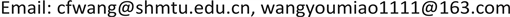﻿ 关于运输问题的几个注记 Some Notes on Transportation Problem

Vol.08 No.04(2018), Article ID:25735,6 pages
10.12677/AE.2018.84055

Some Notes on Transportation Problem

Caifang Wang1, Youmiao Wang2

1College of Arts and Sciences, Shanghai Maritime University, Shanghai

2College of Transport and Communications, Shanghai Maritime University, ShanghaiReceived: Jun. 11th, 2018; accepted: Jun. 26th, 2018; published: Jul. 3rd, 2018ABSTRACT

Transportation problem with balanced production and marketing is an important part of operations research course. This kind of problem can be solved by table dispatching method on a transportation simplex tableau. After explaining the basic methods in class, three questions are put forward for students to think and discuss. 1) When using the potential method to solve the test number of nonbasic variables, the potentials are not unique. Why is the test number unique? 2) How to modify the table dispatching method on the transportation simplex tableau when we want to maximize the objective function of transportation problem? 3) If we use the Lagrange multiplier method to solve this problem, what is the relationship between Lagrange multiplier and potentials? Through the discussion of these problems, students can learn to think and understand.

Keywords:Transportation Problem, Test Number, Potential, Lagrange Multiplier, Karush-Kuhn-Tucker Condition.

1上海海事大学，文理学院，上海

2上海海事大学，交通运输学院，上海1. 运输问题回顾

${x}_{ij}$ 为从产地 ${A}_{i}$ 运往销地 ${B}_{j}$ 的运输量，得到一般运输量问题的模型：

$\mathrm{min}f=\sum _{i=1}^{m}\sum _{j=1}^{n}{c}_{ij}{x}_{ij},$ (1)

$\text{s}\text{.t}\text{.}\left\{\begin{array}{ll}\sum _{j=1}^{n}{x}_{ij}={a}_{i},\hfill & i=1,2,\cdots ,m.\hfill \\ \sum _{i=1}^{m}{x}_{ij}={b}_{j},\hfill & j=1,2,\cdots ,n\text{.}\hfill \\ {x}_{ij}\ge 0,\hfill & i=1,2,\cdots ,m,j=1,2,\cdots ,n.\hfill \end{array}$

2. 主要问题讨论

2.1. 采用位势法计算检验数

2.1.1. 闭回路与非基变量的检验数Figure 1. Closed loop

${\sigma }_{ij}={c}_{ij}-\left({c}_{ik}-{c}_{lk}+{c}_{ls}-{c}_{ts}+{c}_{tj}\right).$ (2)

2.1.2. 位势法计算检验数

${u}_{i}+{v}_{j}={c}_{ij},\text{ }\left(i=1,2,\cdots ,m;j=1,2,\cdots ,n\right)$ (3)

${\sigma }_{ij}={c}_{ij}-\left({u}_{i}+{v}_{j}\right),$ (4)

$\begin{array}{c}{\sigma }_{ij}={c}_{ij}-\left({u}_{i}+{v}_{j}\right)\\ ={c}_{ij}-\left({u}_{i}+{v}_{k}-{v}_{k}-{u}_{l}+{u}_{l}+{v}_{s}-{v}_{s}-{u}_{u}+{u}_{u}+{v}_{j}\right)\\ ={c}_{ij}-\left[\left({u}_{i}+{v}_{k}\right)-\left({u}_{l}+{v}_{k}\right)+\left({u}_{l}+{v}_{s}\right)-\left({u}_{t}+{v}_{s}\right)+\left({u}_{t}+{v}_{j}\right)\right]\\ ={c}_{ij}-\left({c}_{ik}-{c}_{lk}+{c}_{ls}-{c}_{ts}+{c}_{tj}\right).\end{array}$ (5)

2.2. 极大化问题与表上作业法

$\mathrm{max}f=\sum _{i=1}^{m}\sum _{j=1}^{n}{c}_{ij}{x}_{ij},$ (6)

$\text{s}\text{.t}\text{.}\left\{\begin{array}{ll}\sum _{j=1}^{n}{x}_{ij}={a}_{i},\hfill & i=1,2,\cdots ,m.\hfill \\ \sum _{i=1}^{m}{x}_{ij}={b}_{j},\hfill & j=1,2,\cdots ,n.\hfill \\ {x}_{ij}\ge 0,\hfill & i=1,2,\cdots ,m,j=1,2,\cdots ,n.\hfill \end{array}$

$\mathrm{min}z=\sum _{i=1}^{m}\sum _{j=1}^{n}\left(M-{c}_{ij}\right){x}_{ij}=\sum _{i=1}^{m}\sum _{j=1}^{n}\left(-{c}_{ij}\right){x}_{ij}+M\sum _{i=1}^{m}{a}_{i}.$ (7)

$M-{c}_{ij}\ge 0$ 作为极小化问题的单位运价，用原有的表上作业法求出最优解。

1) 初始化：采用最大元素法或其他方法给出初始可行解；

2) 判断当前可行解是否是最优解；采用闭回路法或是位势法对表上空格处的检验数，当所有空格处的检验数

${\sigma }_{ij}={c}_{ij}-{C}_{B}{B}^{-1}{P}_{ij}\le 0$

3) 更新基变量与基解。

a) 选出 ${\sigma }_{rq}>0$ 中的最大值

${\sigma }_{ij}={\mathrm{max}}_{r,q}\left\{{\sigma }_{rq}|{\sigma }_{rq}>0\right\}.$

${x}_{ij}$ 为入基变量。

b) 在运输表上找一条包含 ${x}_{ij}$ 为顶点的闭回路，这条闭回路上的其他顶点皆为基变量(格)。

c) 计算

$\theta =\mathrm{min}\left\{{x}_{rq}|{x}_{rq}对应闭回路上的偶点格\right\}={x}_{ls}称为\theta 的调整量.$

${z}^{\text{old}}=\underset{\begin{array}{c}{x}_{rq}为基变量\\ 且不在闭回路上\end{array}}{\sum \sum }{c}_{rq}{x}_{rq}+\left[{c}_{ik}{x}_{ik}+{c}_{lk}{x}_{lk}+{c}_{ls}{x}_{ls}+{c}_{us}{x}_{us}+{c}_{uj}{x}_{uj}\right].$

$\begin{array}{c}{z}^{\text{new}}=\underset{\begin{array}{c}{x}_{rq}为基变量\\ 且不在闭回路上\end{array}}{\sum \sum }{c}_{rq}{x}_{rq}+\left[{c}_{ik}\left({x}_{ik}-\theta \right)+{c}_{lk}\left({x}_{lk}-\theta \right)+{c}_{ls}\left({x}_{ls}-\theta \right)+{c}_{us}\left({x}_{us}+\theta \right)+{c}_{uj}\left({x}_{uj}-\theta \right)+{c}_{ij}\theta \right]\\ =\underset{\begin{array}{c}{x}_{rq}为基变量\\ 且不在闭回路上\end{array}}{\sum \sum }{c}_{rq}{x}_{rq}+\left[{c}_{ik}{x}_{ik}+{c}_{lk}{x}_{lk}+{c}_{ls}{x}_{ls}+{c}_{us}{x}_{us}+{c}_{uj}{x}_{uj}\right]+\left({c}_{ij}-{c}_{ik}+{c}_{lk}-{c}_{ls}+{c}_{ts}-{c}_{tj}\right)\theta \\ ={z}^{\text{old}}+{\sigma }_{ij}\theta \ge {z}^{\text{old}}.\end{array}$

2.3. 表上作业法与Karush-Kuhn-Tucker条件

$L\left(X;\lambda ,\mu ,d\right)=\sum _{i=1}^{m}\sum _{j=1}^{n}{c}_{ij}{x}_{ij}+\sum _{i=1}^{m}{\lambda }_{i}\left(\sum _{j=1}^{n}{x}_{ij}-{a}_{i}\right)+\sum _{j=1}^{n}{\mu }_{j}\left(\sum _{i=1}^{m}{x}_{ij}-{b}_{j}\right)+\sum _{i=1}^{m}\sum _{j=1}^{n}{d}_{ij}\left(-{x}_{ij}\right).$ (8)

1) 等式约束对应的Lagrange乘子为位势的相反数： ${\lambda }_{i}=-{u}_{i}$${\mu }_{j}=-{v}_{j}$

2) 非负性约束对应的Lagrange乘子为非基变量的检验数： ${d}_{ij}={c}_{ij}+{\lambda }_{i}+{\mu }_{j}={c}_{ij}-\left({u}_{i}+{v}_{j}\right).$

$\frac{\partial L}{\partial {\lambda }_{i}}=\sum _{j=1}^{n}{x}_{ij}^{*}-{a}_{i}=0,$

${x}_{ij}\ge 0,$

$\mathrm{max}w=\sum _{i=1}^{m}{a}_{i}{u}_{i}+\sum _{j=1}^{n}{b}_{j}{v}_{j},$ (9)

$\text{s}\text{.t}\text{.}\text{\hspace{0.17em}}\left\{\begin{array}{l}{u}_{i}+{v}_{j}\le {c}_{ij},\\ {u}_{i},{v}_{j}无符号限制.\end{array}$

$\left(-{\lambda }_{i}\right)+\left(-{\mu }_{j}\right)={c}_{ij}-{d}_{ij}\le {c}_{ij}.$ (10)

$-{\lambda }_{i}$$-{\mu }_{j}$ 为对偶问题1.1的可行解。

$\left(-{\lambda }_{i}-{\mu }_{j}\right){x}_{ij}^{*}=\left({c}_{ij}-{d}_{ij}\right){x}_{ij}^{*}={c}_{ij}{x}_{ij}^{*}.$ (11)

$\sum _{i=1}^{m}\sum _{j=1}^{n}\left(-{\lambda }_{i}-{\mu }_{j}\right){x}_{ij}^{*}=\sum _{i=1}^{m}\sum _{j=1}^{n}{c}_{ij}{x}_{ij}^{*},$ (12)

$\sum _{i=1}^{m}\left(-{\lambda }_{i}\right)\sum _{j=1}^{n}{x}_{ij}^{*}+\sum _{j=1}^{n}\left(-{\mu }_{j}\right)\sum _{i=1}^{m}{x}_{ij}^{*}=\sum _{i=1}^{m}\sum _{j=1}^{n}{c}_{ij}{x}_{ij}^{*}.$ (13)

$\sum _{i=1}^{m}{a}_{i}\left(-{\lambda }_{i}\right)+\sum _{j=1}^{n}{b}_{j}\left(-{\mu }_{j}\right)=\sum _{i=1}^{m}\sum _{j=1}^{n}{c}_{ij}{x}_{ij}^{*}.$ (14)

${x}_{ij}$ 为基变量时， ${d}_{ij}=0$ ，则 $-{\lambda }_{i}-{\mu }_{j}={c}_{ij}$ ，根据位势的定义(3)， $-{\lambda }_{i}$$-{\mu }_{j}$ 为位势；

${x}_{ij}$ 为非基变量时， ${d}_{ij}={c}_{ij}+{\lambda }_{i}+{\mu }_{j}$ 恰为检验数。

3. 总结

Some Notes on Transportation Problem[J]. 教育进展, 2018, 08(04): 364-369. https://doi.org/10.12677/AE.2018.84055

1. 1. 运筹学教材编写组. 运筹学[M]. 第四版. 北京: 清华大学出版社, 2012.

2. 2. 刁在筠, 等. 运筹学[M]. 第三版. 北京: 高等教育出版社, 2007.

3. 3. 施光燕, 钱伟懿, 庞丽萍. 最优化算法[M]. 第二版. 北京: 高等教育出版社, 2007.

4. 4. 朱德通. 最优化模型与实验[M]. 上海: 同济大学出版社, 2003.

5. 5. Nocedal, J., Wright, S.J., Mikosch, T.V., Resnick, S.I. and Robinson, S.M., eds. (2006) Numerical Optimization. Springer Series in Operations Research and Financial Engineering. 2nd Edition, Springer, New York, NY, USA.